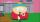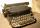Trapezium

The length of the base and the height size of the base of the trapezium is at ratio 5:3:2, the content area of the trapezium is 128 cm2. Calculate the length of the base and the height of the trapezoid.

Result

a =  18.856
c =  11.314
v =  7.542

Solution:Leave us a comment of example and its solution (i.e. if it is still somewhat unclear...):Be the first to comment!To solve this example are needed these knowledge from mathematics:

Looking for help with calculating roots of a quadratic equation? Do you have a linear equation or system of equations and looking for its solution? Or do you have quadratic equation?

Next similar examples:

1. AceThe length of segment AB is 24 cm and the point M and N divided it into thirds. Calculate the circumference and area of this shape.
2. Roof tilesThe roof has a trapezoidal shape with bases of 15 m and 10 m, height of roof is 4 meters. How many tiles will need if on 1 m2 should be used 8 tiles?
3. TrapezoidArea of trapezoid is 135 cm2. Sides a, c and height h are in a ratio 6:4:3. How long are a,c and h? Make calculation...
4. Liters od milkThe cylinder-shaped container contains 80 liters of milk. Milk level is 45 cm. How much milk will in the container, if level raise to height 72 cm?
5. Fifth of the numberThe fifth of the number is by 24 less than that number. What is the number?
6. QuizQ2The square of the first number is equal to three-fifths of the second number. Determine both numbers if you know that the second number is 5 times greater than the first, and neither of numbers is not equal to zero.
7. Copy typistTypist for 12 hours rewritten 15% of the manuscript. After how many hours he will done 35% of the manuscript?
8. FractionFor what x expression ? equals zero?Product of two numbers is 900. If we increase lowest number by 2 then product will increase by 150. Determine both numbers.
10. Dining roomThe dining room has 11 tables (six and eight seats). In total there are 78 seats in the dining room. How many are six-and eight-seat tables?
11. CompetitorsIn the first round of slalom fell 15% of all competitors and in the second round another 10 racers. Together, 40% of all competitors fell. What was the total number of competitors?
12. PupilsThere are 350 girls in the school, and the other 30% of the total number of pupils are boys. How many pupils does the school have?
13. Cinema ticketsCinema sold 180 tickets this Thursday, which is 20%. Monday 14%, Tuesday 6%, Wednesday 9%, Friday 24%, Saturday 12%, and Sunday 15%. How many tickets were sold per week?
14. CandyPeter had a sachet of candy. He wanted to share with his friends. If he gave them 30 candies, he would have 62 candies. If he gave them 40 candies, he would miss 8 candies. How many friends did Peter have?
15. Football seasonDalibor and Adam together scored 97 goals in the season. Adam scored 9 goals more than Dalibor. How many goals scored each?
16. SummerjobThree students participated in the summerjob. Altogether they earn 1780, -. Peter got a third less than John and Paul got 100,- more than Peter. How much got every one of them?
17. ProductThe product of two consecutive odd numbers is 8463. What are this numbers?